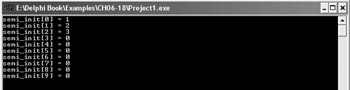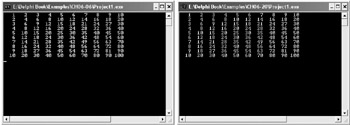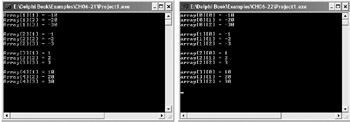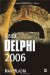Arrays in C

Arrays in C++

Array declaration in C++ is completely different from array declaration in Delphi. In Delphi, you specify the index of the first and the last elements in the array, which defines the number of elements in the array. In C++, you specify the number of elements in the array, and get the index of the first and last element from this number. The index of the first element in a C++ array is always 0, and the index of the last element is always declared_number_of_elements –1.

Here's how to declare an array in C++:

data_type array_name[number_of_elements];

The following example shows how to declare and use an array of 10 integers.

Listing 6-17: C++ arrays#include <iostream.h> #include <conio.h> #pragma hdrstop #pragma argsused int main(int argc, char* argv[]) {    int arr;    int i;    for(i=0; i<10; i++)    {       arr[i] = (i + 1) * 10;       cout << arr[i] << endl;    }    getch();    return 0; }Initializing Arrays

In Delphi, you can initialize global arrays by using the standard array declaration syntax followed by an assignment operator and a comma-delimited list of values enclosed in parentheses (the number of values inside the parentheses must match the number of elements in the array):

var   InitArray: array[1..5] of Integer = (10, 20, 30, 40, 50);

To initialize an array in C++, you have to do almost the same thing as in Delphi with one difference: The comma-delimited list of values must be surrounded by curly braces:

int initialized_array = {1, 2, 3};

In C++, you don't have to explicitly define the number of elements in the array if you're initializing it. In this case, the initialization part of the array declaration defines the number of elements in the array:

data_type array_name[] = {value_1, value_2, value_n};

For instance, the following example shows how you can declare and initialize an array with six elements:

int initialized_array[] = {10, 20, 30, 40, 50, 60};

When you declare an array without explicitly defining the number of elements, you might need to know how many elements there are. To do so, you'll need to use the sizeof operator. When you pass an array to the sizeof operator, it will return the number of bytes occupied by the entire array. So, to get the number of elements in the array, divide the size of the entire array by the size of its first element. The following example shows how to use the sizeof operator to determine the number of elements in the array.

Listing 6-18: Using the sizeof operator to determine the number of elements in an array#include <iostream.h> #include <conio.h> #pragma hdrstop #pragma argsused int main(int argc, char* argv[]) {    int initialized_array[] = {10, 20, 30, 40, 50, 60};    // display the size of the array    cout << "The array occupies " << sizeof(initialized_array)       << " bytes." << endl;    // display the size of each element    cout << "Each element occupies " << sizeof(initialized_array)       << " bytes." << endl;    // display the number of elements    cout << "Number of elements in the array: "       << sizeof(initialized_array) / sizeof(initialized_array)       << endl;    getch();    return 0; }Delphi is much stricter when it comes to array initialization because you have to specify the number of elements and initialize all array elements. In C++, if you explicitly specify the number of elements in the array, you can initialize all of them or just as many as you need. For instance, the following example shows how to declare an array of 10 integers and initialize only the first three. When you initialize only some of the elements in an array, those that aren't initialized are set to 0 by the compiler (see Figure 6-1).Figure 6-1: Initializing arrays in C++

Listing 6-19: Initializing only some of the elements in an array#include <iostream.h> #include <conio.h> #pragma hdrstop #pragma argsused int main(int argc, char* argv[]) {    int semi_init = {1, 2, 3};    for(int i=0; i<10; i++)       cout << "semi_init[" << i << "] = " <<  semi_init[i] << endl;    getch();    return 0; }Multidimensional Arrays

To declare a multidimensional array in C++, you have to write this:

data_type array_name[n1][n2];

The first number is the number of one-dimensional arrays and the second number is the number of elements in each one-dimensional array:

// ten arrays with 5 elements each int multi_array; // find out the number of elements in each array cout << sizeof(multi_array) / sizeof(multi_array) << endl;

The following example shows how to build a multiplication table and store it into a two-dimensional array. Listing 6-6 shows the Delphi implementation of this example.

Listing 6-20: Using a two-dimensional array in C++#include <iostream.h> #include <conio.h> #pragma hdrstop #pragma argsused int main(int argc, char* argv[]) {    int mult_table;    for(int i=0; i<10; i++)    {       for(int j=0; j<10; j++)       {         mult_table[i][j] = (i+1) * (j+1);         cout.width(4);         cout << mult_table[i][j];       }       cout << endl;    }    getch();    return 0; }In the Delphi example, the Write(data:width) syntax was used to specify the width of each item displayed on screen. To specify the width of an item in C++, you have to write cout.width(width).

Both Write(data:4) and cout.width(4) result in columns that are four characters wide. Figure 6-2 shows both the Delphi (left) and the C++ (right) multiplication table examples.Figure 6-2: Specifying the display width in Delphi and C++ console applications

Initializing Multidimensional Arrays

In Delphi, you can initialize global multidimensional arrays or declare multidimensional constant arrays using the following syntax:

ArrayName: array[index_1, index_2] of DataType = (      (1st_array_val_1, 1st_array_val_2, 1st_array_val_n),      (2nd_array_val_1, 2nd_array_val_2, 2nd_array_val_n)     // etc.. );

The example in Listing 6-21 shows how to initialize a global multidimensional array in Delphi and display its values on screen.

Listing 6-21: Initializing a multidimensional array in Delphiprogram Project1; {\$APPTYPE CONSOLE} uses   SysUtils; var   i, j: Integer;   MultiInit: array[1..4, 1..3] of Integer = (      (-10, -20, -30),      (-1, -2, -3),      (1, 2, 3),      (10, 20, 30)   ); begin   for i := Low(MultiInit) to High(MultiInit) do   begin     for j := Low(MultiInit) to High(MultiInit) do     begin       WriteLn('Array[', i, '][', j, ']', ' = ',  MultiInit[i,j]);     end;     WriteLn;   end;   ReadLn; end.In C++, you can initialize a multidimensional array like you would a one-dimensional array and you can initialize it as you would initialize it in Delphi, with each array's values in separate parentheses (in C++, parentheses are replaced with curly braces).

The following listing shows both ways of initializing multidimensional arrays. Figure 6-3 shows what Delphi and C++ initialized multidimensional arrays look like.Figure 6-3: Initialized multidimensional arrays

Listing 6-22: Initializing multidimensional arrays in C++#include <iostream.h> #include <conio.h> #pragma hdrstop #pragma argsused int main(int argc, char* argv[]) {    // initialize it as you would a one-dimensional array    // int array1 = {-10, -20, -30, -1, -2, -3, 1, 2, 3, 10, 20, 30};    // multidimensional array initialization, more readable    int array2 = {       {-10, -20, -30},       {-1, -2, -3},       {1, 2, 3},       {10, 20, 30}    };    for(int i=0; i<4; i++)    {       for(int j=0; j<3; j++)       {         cout << "array[" << i << "][" << j << "] = "            << array2[i][j] << endl;       }       cout << endl;    }    getch();    return 0; }Inside Delphi 2006 (Wordware Delphi Developers Library)
ISBN: 1598220039
EAN: 2147483647
Year: 2004
Pages: 212